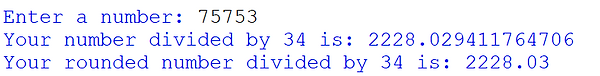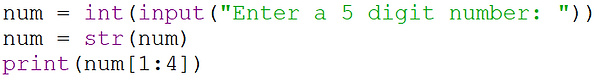top of page
top

## Python 9b - Number Handling## Rounding Numbers

The round() command is used to round a value to a certain number of decimal places.

Type your variable into the round command brackets, add a comma and state the number of decimal places to round to.If you type only the variable, it will round the number to the nearest whole value.

Ask the user to enter any large number.

Ask the user to enter another large number.

Divide the two numbers and print the answer to 3 decimal places.

Example solution:## Using Numbers as Strings

The following techniques all require the integer to be converted into a string first using the str command.

Just like a string, you can shorten a variable to only display a certain length.

Remember that Python starts at zero.You can select a specific digit in the same manner as when selecting characters in a string.If you want to use your variable as an integer again later you would need to convert it from a string to an integer using the int command.

Again, reversing a number is the same as reversing a string.You can also use other string handling methods such as .startswith() or .endswith()

Ask the user to enter a 10 digit number.

Select the 2nd and 8th digits and add them together.

Print the total.

Example solution:bottom of page